International
Tables for
Crystallography
Volume G
Definition and exchange of crystallographic data
Edited by S. R. Hall and B. McMahon

International Tables for Crystallography (2006). Vol. G. ch. 3.3, pp. 118-121

## Section 3.3.4. Experimental measurements

B. H. Tobya*

aNIST Center for Neutron Research, National Institute of Standards and Technology, Gaithersburg, Maryland 20899-8562, USA
Correspondence e-mail: brian.toby@nist.gov

### 3.3.4. Experimental measurements

| top | pdf |

The categories in the powder CIF dictionary relating to the crystallographic experiment are as follows:

 Characterization and preparation of the sample (§3.3.4.1) PD_CHAR group PD_CHAR PD_PREP group PD_PREP Description of the specimen (§3.3.4.2) PD_SPEC group PD_SPEC Instrument calibration and design (§3.3.4.3) PD_CALIB group PD_CALIB PD_CALIBRATION PD_INSTR group PD_INSTR Observations and measurement conditions (§3.3.4.4) PD_DATA group PD_DATA (items beginning with _pd_meas_*) PD_MEAS group PD_MEAS_INFO PD_MEAS_METHOD

The pdCIF dictionary differentiates between the terms sample and specimen. The terms are often treated as interchangeable, but they have quite distinct meanings. The term sample refers to a batch of material, while the term specimen refers to the particular portion of the sample that was used for a measurement. In some cases, the specimen is modified before it is used for data collection. For example, it may be mixed with an internal standard, dried, hydrated or pressed into a pellet.

#### 3.3.4.1. Characterization and preparation of the sample

| top | pdf |

The data items in these categories are as follows:

(a) PD_CHAR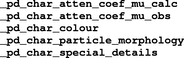(b) PD_PREP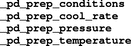The PD_CHAR data items describe information known about the sample from observation and chemical analysis. For example, a description of the sample morphology can be specified using _pd_char_particle_morphology. Note that there are data items in the core dictionary that are appropriate for use with powder diffraction. For example, _atom_type_analytical_mass_% can be used for chemical analysis results and _chemical_melting_point for the melting point. Several similar data items occur in the pdCIF and core dictionaries. _exptl_crystal_colour and _pd_char_colour both describe the sample colour, but _pd_char_colour is more systematic. Also, _pd_char_atten_coef_mu_calc and _exptl_absorpt_coefficient_mu describe similar properties, but _pd_char_atten_coef_mu_calc is adjusted for the sample packing fraction, so it can be compared with the experimental value, _pd_char_atten_coef_mu_obs, when a direct measurement is made.

The PD_PREP data items describe how the sample was collected or prepared. For example, _pd_prep_pressure and _pd_prep_temperature describe the pressure and temperature used to prepare the sample. Note that these will probably differ from the pressure and temperature conditions at which diffraction measurements are made. Measurement conditions are recorded in _diffrn_ambient_pressure and _diffrn_ambient_temperature.

#### 3.3.4.2. Description of the specimen used in the experiment

| top | pdf |

The data items in this category are as follows:

PD_SPECThe PD_SPEC data items describe the specimen used to measure the diffraction data. The data item _pd_spec_preparation describes how the specimen that was used to measure the diffraction data was treated, not how the sample was prepared (PD_PREP) or characterized ( PD_CHAR).

The PD_SPEC data items are also used to describe how the specimen was mounted for the diffraction experiment. For example, _pd_spec_mount_mode and _pd_spec_orientation describe the measurement geometry, while _pd_spec_shape and _pd_spec_size_* describe the specimen shape and size.

#### 3.3.4.3. Instrument calibration and description

| top | pdf |

The data items in these categories are as follows:

(a) PD_CALIB(b) PD_CALIBRATION(c) PD_INSTR(d) Part of PD_DATA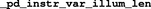The bullet () indicates a category key.

Calibration information can be placed in the PD_CALIB and PD_CALIBRATION categories. The _pd_calibration_* data items are descriptive and will not appear in a loop. The _pd_calib_* items may be looped to describe multiple detectors. Correction values forcan be given using the _pd_calib_2theta_offset and _pd_calib_2theta_off_* data items. A calibration equation can be given using _pd_calibration_conversion_eqn. When multiple detectors are used, _pd_calib_detector_response is used to indicate the relative performance of each detector. The detector deadtime is specified using the core data item _diffrn_detector_dtime (which cannot be looped by detector).

If an internal standard is added to the sample for calibration, this information is specified using _pd_calib_std_internal_name to specify the material added and _pd_calib_std_internal_mass_% to specify the amount.

When a set of calibration intensities is measured using an external standard, it is possible to include the measurements and the derived results in a separate CIF block. A data block would then use _pd_calib_std_external_block_id to link to the block containing the calibration information. See Section 3.3.7for a discussion of block pointers and block IDs. Note that the use of a unique name for the block ID allows the calibration information to be stored in a separate file, so that the calibration CIF need not be repeated in every CIF that references it.

The PD_INSTR section of the pdCIF dictionary contains terms that describe the instrument used. For example, the instrument or laboratory location is given using _pd_instr_location. The instrument type can be indicated using _pd_instr_geometry. The instrument geometry can be described in much greater detail using several data items. The geometry is described in terms of four regions of the experiment: radiation source to monochromator (src/mono); monochromator to specimen (mono/spec); specimen to analyser (spec/anal); and analyser to detector (anal/detc). If no monochromator is present, the first two regions are combined into radiation source to specimen (src/spec). If no analyser is present, the last two regions are combined into specimen to detector (spec/detc). Thus two, three or four sets of values describe the dimensions of the instrument and the collimation. For example, _pd_instr_dist_src/mono would be used to specify the distance between the radiation source and the monochromator. Alternatively, _pd_instr_dist_src/spec would be used to specify the distance between the radiation source and the specimen if no monochromator was present.

Two methods may be used to describe the slits limiting the divergence in the equatorial plane. The angular divergence allowed by the slits in degrees can be specified using the _pd_instr_divg_eq_* data items. Alternatively, the dimensions of the slits in the equatorial direction in millimetres may be specified using _pd_instr_slit_eq_*. The dimensions of these slits in the axial direction, i.e. the direction perpendicular to the equatorial plane and containing the incident or diffracted beam as appropriate, are specified in millimetres using _pd_instr_slit_ax_*. The axial slit lengths, along with the _pd_instr_dist_* distances, are useful for estimating the low-angle peak asymmetry (Finger et al., 1994). Note that angular divergence in the axial plane is not a well defined concept for line-focus instruments, but can be specified, where appropriate, using _pd_instr_divg_ax_*.

The axial and equatorial directions are shown schematically in Fig. 3.3.4.1. The equatorial plane contains the equatorial direction vectors, as well as the incident beam, the diffracted beam and the scattering vector. The axial plane is perpendicular to the equatorial plane and contains the sample centre, which is the point where the incident and diffracted beams meet. For area-detection instruments, the designations of axial and equatorial directions may be arbitrary.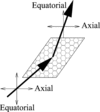Figure 3.3.4.1 | top | pdf |The axial and equatorial directions in a powder-diffraction experiment.

Soller collimators are described using _pd_instr_soller_eq_* data items rather than _pd_instr_divg_eq_* data items. It is common practice to specify the Soller collimation in arc-minutes (e.g. 30′). However, pdCIF defines these items to have units of degrees, so 30′ would be recorded in the CIF as 0.5. It is not usual to limit the axial divergence, except to reduce low-angle asymmetry, but if this is done, the _pd_instr_soller_ax_* data items can be used to define this.

For constant-wavelength instruments, it is common to have a monochromator or filter either before the sample, or after the sample (an analyser), or sometimes both. This is described using _pd_instr_monochr_pre_spec and _pd_instr_monochr_post_spec. It is rare, but possible, to have both a filter and a monochromator in the same location. The BT-1 neutron powder diffractometer at NIST uses both a Cu(311) monochromator and a graphite filter to attenuate thecomponent. In this case, the two elements would be placed in a loop: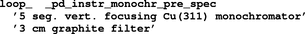Note that the monochromator and analyser takeoff angles are given using _pd_instr_2theta_monochr_pre and _pd_instr_2theta_monochr_post. It is useful to record these values for X-ray studies, as they are needed for proper polarization corrections.

In a conventional Bragg–Brentano diffractometer, the divergence slits limit the illumination area at the sample. However, since theaxis (the sampleaxis) is usually set to bisect theangle of the detector, the actual length of the area of the sample that is illuminated changes with. One should choose divergence slits so that the beam does not illuminate areas outside the sample at the lowest diffraction angle used. An alternative method for data collection is to have a divergence slit that opens asincreases, so that a constant area of the sample is illuminated. This is known as a-compensating slit. Using a-compensating slit provides a better signal-to-noise ratio at largervalues, but means that the diffraction intensities have to be normalized to compensate for the change in illumination. It also introduces greater optical aberrations with; a flat plate becomes an increasingly worse approximation to the curved sample geometry in the true Bragg–Brentano geometry.

The use of a variable divergence slit can be recorded in the form: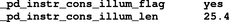Note that if _pd_instr_cons_illum_flag is not specified, the value is assumed to be no, indicating that a fixed-width divergence slit has been used.

The beam size can be specified in two different ways: as the size at the source, using _pd_instr_source_size_ax and _pd_instr_source_size_eq, or as the size at the sample position, using _pd_instr_beam_size_ax and _pd_instr_beam_size_eq. Note that the size of the beam at the sample differs from the illumination length described above except when the sample is perpendicular to the beam. When a variable-divergence slit is in use, the beam size at the sample changes with, so if this size is known directly, the _pd_instr_beam_size_eq data item can be included in the loop containing the diffraction intensities. Similarly, in a constant-divergence instrument, where the illumination length changes with, the illumination length can be specified in the loop using _pd_instr_var_illum_len.

There are also several data items in the core CIF dictionary that should be present in the description of the instrument in a pdCIF. Use _diffrn_radiation_probe and _diffrn_radiation_type to specify the type of radiation used and _diffrn_detector_type to specify the detection type.

#### 3.3.4.4. Observations and measurement conditions

| top | pdf |

The data items in these categories are as follows:

(a) Part of PD_DATA(b) PD_MEAS_INFO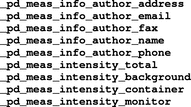(c) PD_MEAS_METHOD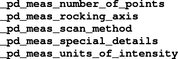The arrow () is a reference to a parent data item.

The item _pd_data_point_id identifies each entry in the list of measured, processed or simulated intensities. It is the only item in the PD_DATA category that actually begins with the string _pd_data_, and is included here for convenience. If the list of intensities is split across several distinct loops, the role of this identifier may be adopted by other identifiers, such as _pd_meas_point_id in an isolated list of measured intensities.

The _pd_meas_* data items contain unprocessed measurements and documentation on the instrumental settings used for the measurements. Note that the choice of the data items used to represent this information is determined by the type of diffraction instrument, as well as how the measurement was conducted. This will be discussed further in Section 3.3.8. However, some _pd_meas_* data items are independent of the instrument type. For example, the use of _pd_meas_datetime_initiated is good practice, as is use of the _pd_meas_info_author_* data items. It is probably good practice to record the number of data points in _pd_meas_number_of_points for the benefit of people who might read the CIF, but there is no requirement that this item be present. This means that software should determine the number of data points directly when reading the CIF, rather than relying on the presence of a value for _pd_meas_number_of_points.

### ReferencesFinger, L. W., Cox, D. E. & Jephcoat, A. P. (1994). A correction for powder diffraction peak asymmetry due to axial divergence. J. Appl. Cryst. 27, 892–900.Google Scholar